GRE Subject Test: Chemistry : Orbitals and Orbital Hybridization

Example Questions

Example Question #1 : Nuclear Chemistry

What hybrid orbitals are found in a molecule of water?

sp3

sp

sp3d

sp2

sp3

Explanation:

The hybrid orbitals for a molecule are determined by the number of atoms and lone pairs attached to the central atom. These two numbers are added to each other in order to determine the hybridization of the bonds. If there are two atoms or lone pairs attached to the central atom, it will exhibit sp hybridization. If there are three total atoms or lone pairs attached to the central atom, it will display sp2 hybridization. Water has two hydrogens and two lone pairs attached. This means that water has sp3 hybridization.

Example Question #1 : Orbitals And Orbital HybridizationWhat is the hybridization of the indicated carbon in the given molecule?

sp

sp3

spf

sp2d

sp2

sp3

Explanation:

The correct answer is sp3. First knock out any answers that aren't actual orbitals (for example spf skipped the d orbital entirely). After doing this, tackle the problem using the following guidelines:

Electrons are contained within orbitals that carry different names (s, p, d, f) based on how far they are from the nucleus. The further these atomic orbitals are away from the nucleus, the higher in energy they are. When two atoms come together to form a covalent bond, their outer most orbitals overlap and the electrons within them are shared. Two s atomic orbitals overlapping would be lower energy (and thus more stable) than say if an s and a p orbital or two p orbitals overlapped.

The geometry associated with compounds and the strengths of each bond would then be varied based on which atomic orbitals decided to overlap, but in simple molecules like methane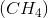the bond strengths are all equivalent and the molecular geometry is quite uniform/precise. It was theorized that all the orbitals involved in bonding might merge and form new shapes called hybrid orbitals. This allowed all the hybrid orbitals to obtain the same energy level as seen in nature. So if an s atomic orbital and a p atomic orbital combined, they would form an sp hybrid orbital with combined properties of both those atomic orbitals.

When determining how many atomic orbitals are being used in covalent bonds for an atom within a molecule, you first count how many sigma (single) bonds there are and ignore any pi (double) bonds. If there are 3 sigma bonds, that means you have s + p + p atomic orbitals combining to form 3 sp3 hybrid orbitals (and so the hybridization of that atom would be sp3). We ignore pi (double) bonds because they are formed by the overlap to 2 p atomic orbitals. Also don’t forget to count invisible hydrogens that are not always drawn in.

Example Question #2 : Nuclear ChemistryWhat is the hybridization of the carbon being pointed to by the arrow?

sp3d

sp3df

sp

sp2

sp3

sp3

Explanation:

The correct answer is sp3. First knock out any answers that make no sense for carbon based upon its valency (aka carbon doesn't have access to higher energy orbitals like d or f). After doing this you can tackle the problem using the following rules.

Hybridization theory confuses a lot of people. Here is the main concept quickly summed up. Electrons are contained within orbitals which carry different names (s, p, d, f) based upon how far they are from the nucleus. The further these atomic orbitals are away from the nucleus, the higher in energy they are. When two atoms come together to form a covalent bond, their outer most orbitals overlap and the electrons within them are shared. Two s atomic orbitals overlapping would be lower energy (and thus more stable) than say if an s and a p orbital or two p orbitals overlapped.

The geometry associated with compounds and the strengths of each bond would then be varied based on which atomic orbitals decided to overlap, but in simple molecules like methane (CH4) the bond strengths are all equivalent and the molecular geometry is quite uniform/precise. It was theorized that all the orbitals involved in bonding might merge and form new shapes called hybrid orbitals. This allowed all the hybrid orbitals to obtain the same energy level as seen in nature. So if an s atomic orbital and a p atomic orbital combined, they would form an sp hybrid orbital with combined properties of both those atomic orbitals.

When determining how many atomic orbitals are being used in covalent bonds for an atom within a molecule, you first count how many sigma (single) bonds there are and ignore any pi (double) bonds. If there are 3 sigma bonds, that means you have s + p + p atomic orbitals combining to form 3 sp3 hybrid orbitals (and so the hybridization of that atom would be sp3). We ignore pi (double) bonds because they are formed by the overlap to 2 p atomic orbitals. Also don’t forget to count invisible hydrogens that are not always drawn in!!! With these few rules you should never get a hybridization question wrong.

Example Question #61 : Gre Subject Test: ChemistryWhat is the hybridization of the indicated carbon in the given molecule?

sp

sp3d

sp2

sp3

sppp

sp2

Explanation:

The correct answer is sp2. First knock out any answers that aren't actual orbitals (for example sppp is not the proper notation so you could knock that out as an answer choice). After doing this you can tackle the problem using the following summary:

Electrons are contained within orbitals that carry different names (s, p, d, f) based on how far they are from the nucleus. The further these atomic orbitals are away from the nucleus, the higher in energy they are. When two atoms come together to form a covalent bond, their outer most orbitals overlap and the electrons within them are shared. Two s atomic orbitals overlapping would be lower energy (and thus more stable) than say if an s and a p orbital or two p orbitals overlapped.

The geometry associated with compounds and the strengths of each bond would then be varied based on which atomic orbitals decided to overlap, but in simple molecules like methanethe bond strengths are all equivalent and the molecular geometry is quite uniform/precise. It was theorized that all the orbitals involved in bonding might merge and form new shapes called hybrid orbitals. This allowed all the hybrid orbitals to obtain the same energy level as seen in nature. So if an s atomic orbital and a p atomic orbital combined, they would form an sp hybrid orbital with combined properties of both those atomic orbitals.

When determining how many atomic orbitals are being used in covalent bonds for an atom within a molecule, you first count how many sigma (single) bonds there are and ignore any pi (double) bonds. If there are 3 sigma bonds, that means you have s + p + p atomic orbitals combining to form 3 sp3 hybrid orbitals (and so the hybridization of that atom would be sp3). We ignore pi (double) bonds because they are formed by the overlap to 2 p atomic orbitals. Also don’t forget to count invisible hydrogens that are not always drawn in.

Example Question #62 : Gre Subject Test: ChemistryWhat is the hybridization of the indicated carbon in the given molecule?

sp2

sp

sp3

sdp

sp3d

sp2

Explanation:

The correct answer is sp2. First knock out any answers that aren't actual orbitals (for example sdp has the orbitals out of order of their respective energy levels). After doing this, tackle the problem using the following guidelines:

Electrons are contained within orbitals that carry different names (s, p, d, f) based on how far they are from the nucleus. The further these atomic orbitals are away from the nucleus, the higher in energy they are. When two atoms come together to form a covalent bond, their outer most orbitals overlap and the electrons within them are shared. Two s atomic orbitals overlapping would be lower energy (and thus more stable) than say if an s and a p orbital or two p orbitals overlapped.

The geometry associated with compounds and the strengths of each bond would then be varied based on which atomic orbitals decided to overlap, but in simple molecules like methanethe bond strengths are all equivalent and the molecular geometry is quite uniform/precise. It was theorized that all the orbitals involved in bonding might merge and form new shapes called hybrid orbitals. This allowed all the hybrid orbitals to obtain the same energy level as seen in nature. So if an s atomic orbital and a p atomic orbital combined, they would form an sp hybrid orbital with combined properties of both those atomic orbitals.

When determining how many atomic orbitals are being used in covalent bonds for an atom within a molecule, you first count how many sigma (single) bonds there are and ignore any pi (double) bonds. If there are 3 sigma bonds, that means you have s + p + atomic orbitals combining to form 3 sp2 hybrid orbitals (and so the hybridization of that atom would be sp2). We ignore pi (double) bonds because they are formed by the overlap to 2 p atomic orbitals. Also don’t forget to count invisible hydrogens that are not always drawn in.

*If this is a little confusing to think of at the moment, you can always calculate:

steric number = # of atoms bonded (to the atom in question) + lone pairs on that atom

If the steric number is 4 = sp3, if 3 = sp2, if 2 = sp

Example Question #2 : Orbitals And Orbital HybridizationNote the indicated carbon. What bond angle is this carbon experiencing?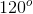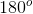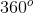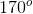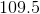Explanation:

The correct answer is. First determine the hybridization of the carbon being pointed to. This carbon is sp hybridized with a steric number of 2. This can be calculated via the following:

steric number = # of atoms bonded (to the atom in question) + lone pairs on that atom. If the steric number is 4 = sp3, if 3 = sp2, if 2 = sp

Next with your understanding of hybridization theory, you know that carbons that are sp hybridized are linear by nature (a common linear molecule example is carbon dioxide). If the geometry of that carbon is linear then that means the bond angles it is experiencing must be.

All GRE Subject Test: Chemistry Resources